Express the polynomial without the cross product terms: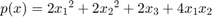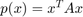, where x is [x_1;x_2,x_3] (in MATLAB notation)

```A = [2 2 0; 2 2 0; 0 0 2];
```

We want to substitute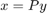so thatis orthogonal. Then, we would get. If we find athat orthogonally diagonalizes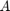, then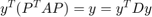, where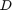is a matrix with the main diagonal containing eigenvalues of.

```[P,D] = eig(A)
```
```P =

-0.7071         0    0.7071
0.7071         0    0.7071
0    1.0000         0

D =

0     0     0
0     2     0
0     0     4

```

We have. So,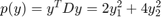.